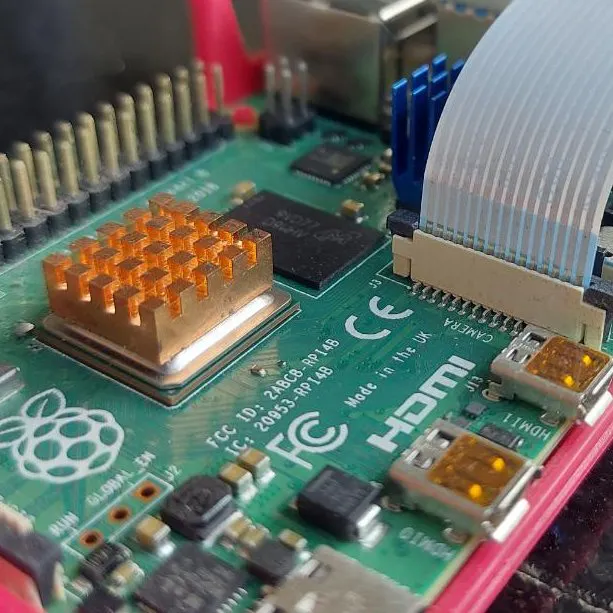Published © GPL3+

# UV disinfection robot

Ultraviolet disinfection remote controlled robot with automatic human detection and collision avoidance.

BeginnerFull instructions providedOver 3 days1,246

## Things used in this project

### Hardware components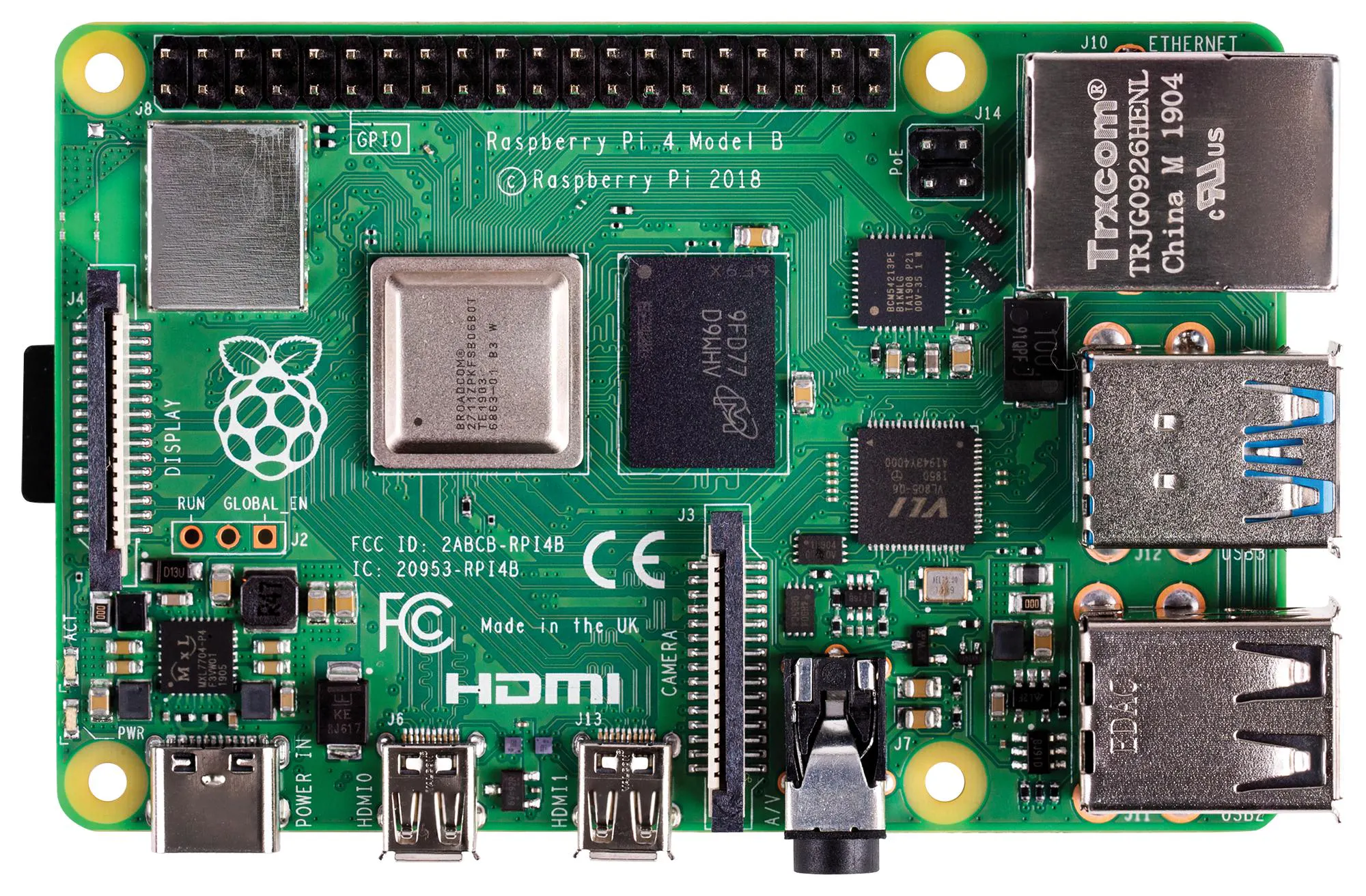Raspberry Pi 4 Model B
×1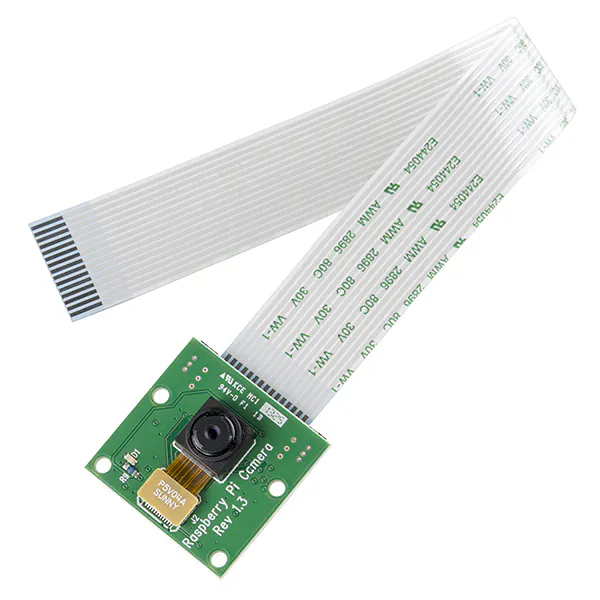Raspberry Pi Camera Module
×1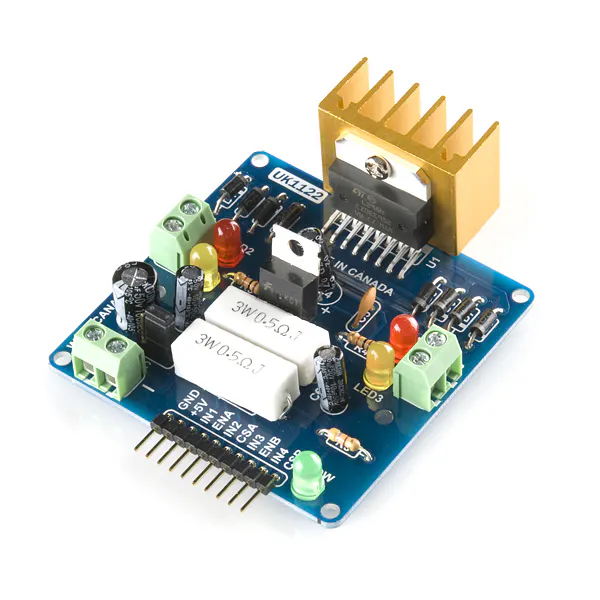SparkFun Dual H-Bridge motor drivers L298
×1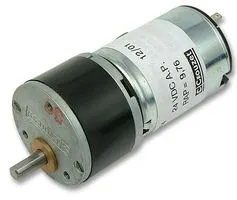Geared DC Motor, 12 V
×4Ultrasonic Sensor - HC-SR04 (Generic)
×2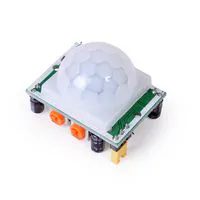PIR Sensor, 7 m
×2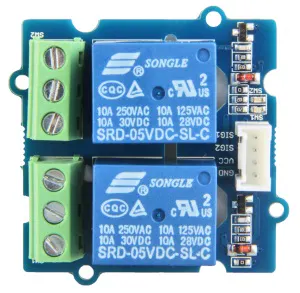Seeed Grove - 2-Channel SPDT Relay
×1Rechargeable Battery, 12 V
×1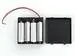4xAA battery holder
×1Jumper wires (generic)
×1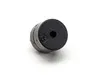Buzzer
×1
 Motor wheel
×4
 UV lamp 11w with Driver 230v
×4
 Inverter 150w
×1
 Thermocol 100cm x 50cm
×2
 Aluminium foil 2m
×1
 PVC pipe 50mm,1m
×1
 Plywood 30cm x 50cm
×1

### Software apps and online servicesRaspberry Pi Raspbian
 VNC viewer
 Mu: IDE You can use any IDEs

### Hand tools and fabrication machinesSoldering iron (generic)Drill / Driver, CordlessHot glue gun (generic)Tape, Electrical

Read more

## Schematics

### UV_Schematic_diagram

The circuit contain all sensors and boards

## Code

### Code for Vision

Python
```from picamera import PiCamera
from time import sleep

def piCam(w=200,h=100,x=0,y=0,fill=True):
camera = PiCamera()
camera.resolution = (w,h)
camera.start_preview(fullscreen= fill,window=(x,y,w,h))
```

### Code for controlling robot

Python
```import pygame

def init():
pygame.init()
win = pygame.display.set_mode((100,100))

def getKey(keyName):
ans = False
for eve in pygame.event.get():pass
keyInput = pygame.key.get_pressed()
myKey = getattr(pygame,'K_{}'.format(keyName))
if keyInput [myKey]:
ans = True
pygame.display.update()

return ans
```

### The main code

Python
In the main code PIR sensor and Relay also included.
```from motor_driver import Motor  #motor driver module
import key_press as kp     #keyboard module for movements of robot and control Uv light
from camera import piCam  #camera module for camera view
import RPi.GPIO as GPIO #gpio module for relay and pir sensor
import ultrasonic as ults #importing ultrasonic modules
GPIO.setmode(GPIO.BCM)
GPIO.setwarnings(False)

motor = Motor(2,3,4,17,22,27) #motor driver pins
runCamera = True
GPIO.setup(14,GPIO.OUT) #defining the relay pin(relay control the UV light)
GPIO.setup(23,GPIO.OUT) #pir uv light control
GPIO.setup(5,GPIO.OUT) #buzzer
GPIO.setup(15,GPIO.IN)  #defining the first pir module
GPIO.setup(18,GPIO.IN)  #defining the second pir module

kp.init() #initialization of keyboard
if runCamera: piCam(x=1,y=1)

def main():

if kp.getKey('UP'): #if press 'UP' key move forward
result = ults.checkFrontDistance(30) # checking the Front limit distance at 30cm
if result== True:
print("moving forward")
GPIO.output(5,GPIO.LOW)
motor.move(0.6,0,0.1) #motor run '60%' of speed with '0'turning and '100ms'delay
else :
print("limit is over")
GPIO.output(5,GPIO.HIGH) #buzzer on
motor.stop(1)
elif kp.getKey('DOWN'):# if press 'DOWN' key the motor move backward
result = ults.checkBackDistance(30)# checking the Back limit distance at 30cm
if result == True:
print("moving backward")
GPIO.output(5,GPIO.LOW)
motor.move(-0.6,0,0.1) #motor run '60%' of speed with '0'turning and '100ms'delay
else :
print("limit is over")
GPIO.output(5,GPIO.HIGH) #buzzer on
motor.stop(1)
elif kp.getKey('LEFT'): #if press 'LEFT' key turn left
result = ults.checkFrontDistance(30)# checking the Front limit distance at 30cm
if result == True:
print("turnng left")
GPIO.output(5,GPIO.LOW)
motor.move(0.6,0.4,0.1) #motor turn at '60%'speed with '0.3' turning and 100ms delay
else:
print("limit is over")
GPIO.output(5,GPIO.HIGH) #buzzer on
motor.stop(1)
elif kp.getKey('RIGHT'): #if press 'RIGHT'key turn right
result = ults.checkFrontDistance(30)# checking the Front limit distance at 30cm
if result == True :
print("turning right")
GPIO.output(5,GPIO.LOW)
motor.move(0.6,-0.5,0.1) #motor run '60%' of speed with '-0.5'turning and '100ms'delay
else:
print("limit is over")
GPIO.output(5,GPIO.HIGH) #buzzer on
motor.stop(1)
elif kp.getKey('q'): #for on
GPIO.output(23,GPIO.LOW) # uv light on
elif kp.getKey('w'): #for off
GPIO.output(23,GPIO.HIGH) #uv light off
elif GPIO.input(15): # when the first pir sensor reads
print("pir 2 ")
GPIO.output(14,GPIO.LOW) # the UV light turn off
GPIO.output(5,GPIO.HIGH) #buzzer on
print("Humen detected")
elif GPIO.input(18): # when the second pir sensor reads
print("pir 1 ")
GPIO.output(14,GPIO.LOW) # the UV light turn off
GPIO.output(5,GPIO.HIGH) #buzzer on
print("Humen detected")

else :
motor.stop(0.2) # else motor delay for 200ms

if __name__ == '__main__': #when run this code the __name__ is return to __main__
while True:
main() # then work the main function
```

### Code for collision avoidance

Python
```import RPi.GPIO as GPIO
import time
GPIO.setmode(GPIO.BCM)
GPIO.setwarnings(False)

def checkFrontDistance(limitation):
TRIG = 20
GPIO.setup(TRIG,GPIO.OUT)
ECHO = 21
GPIO.setup(ECHO,GPIO.IN)
i = 0
verification = False
avgDistance = 0
for i in range(5):
GPIO.output(TRIG, False)
time.sleep(0.1)

GPIO.output(TRIG, True)
time.sleep(0.00001)
GPIO.output(TRIG, False)
while GPIO.input(ECHO)==0:
pulse_start = time.time()

while GPIO.input(ECHO)==1:
pulse_end = time.time()

pulse_duration = pulse_end - pulse_start

distance = pulse_duration * 17150
distance = round(distance,2)
avgDistance=avgDistance+distance

print(" Front Distance" + str(avgDistance))

if avgDistance < limitation:
verification = False
if avgDistance > limitation:
verification = True
return verification

def checkBackDistance(limitation):
TRIG = 8
GPIO.setup(TRIG,GPIO.OUT)
ECHO = 7
GPIO.setup(ECHO,GPIO.IN)
i = 0
verification = False
avgDistance = 0
for i in range(5):
GPIO.output(TRIG, False)
time.sleep(0.1)

GPIO.output(TRIG, True)
time.sleep(0.00001)
GPIO.output(TRIG, False)
while GPIO.input(ECHO)==0:
pulse_start = time.time()

while GPIO.input(ECHO)==1:
pulse_end = time.time()

pulse_duration = pulse_end - pulse_start

distance = pulse_duration * 17150
distance = round(distance,2)
avgDistance=avgDistance+distance

print("Back Distance" + str(avgDistance))

if avgDistance < limitation:
verification = False
if avgDistance > limitation:
verification = True
return verification
```

### Cod for motor driver

Python
```import RPi.GPIO as GPIO
from time import sleep
GPIO.setmode(GPIO.BCM)
GPIO.setwarnings(False)

class Motor():
def __init__(self,EnaA,In1A,In2A,EnaB,In1B,In2B):
self.EnaA = EnaA
self.In1A = In1A
self.In2A = In2A
self.EnaB = EnaB
self.In1B = In1B
self.In2B = In2B
GPIO.setup(self.EnaA,GPIO.OUT)
GPIO.setup(self.In1A,GPIO.OUT)
GPIO.setup(self.In2A,GPIO.OUT)
GPIO.setup(self.EnaB,GPIO.OUT)
GPIO.setup(self.In1B,GPIO.OUT)
GPIO.setup(self.In2B,GPIO.OUT)
self.pwmA = GPIO.PWM(self.EnaA,100)
self.pwmA.start(0)
self.pwmB = GPIO.PWM(self.EnaB,100)
self.pwmB.start(0)

def move(self,speed=0.5,turn=0,t=0):
speed *= 100
turn *= 100
Leftspeed = speed - turn
Rightspeed = speed + turn

if Leftspeed >100: Leftspeed = 100
elif Leftspeed <-100: Leftspeed = -100
if Rightspeed >100: Rightspeed = 100
elif Rightspeed <-100: Rightspeed =-100

self.pwmA.ChangeDutyCycle(abs(Leftspeed))
self.pwmB.ChangeDutyCycle(abs(Rightspeed))

if Leftspeed>0:
GPIO.output(self.In1A,GPIO.HIGH)
GPIO.output(self.In2A,GPIO.LOW)
else :
GPIO.output(self.In1A,GPIO.LOW)
GPIO.output(self.In2A,GPIO.HIGH)

if Rightspeed>0:
GPIO.output(self.In1B,GPIO.HIGH)
GPIO.output(self.In2B,GPIO.LOW)
else :
GPIO.output(self.In1B,GPIO.LOW)
GPIO.output(self.In2B,GPIO.HIGH)

sleep(t)

def stop(self,t=0):
self.pwmA.ChangeDutyCycle(0)
self.pwmB.ChangeDutyCycle(0)
sleep(t)

def main():
motor1.move(0.6,0,0.1)
motor1.stop(2)
motor1.move(0.6,0,0.1)
motor1.stop(2)

if __name__ == '__main__':
motor1 = Motor(2,3,4,17,22,27)
main()
```

## Credits

### fulail

3 projects • 3 followers
Arduino Raspberry pi

### Hafiz Suhail

0 projects • 0 followers